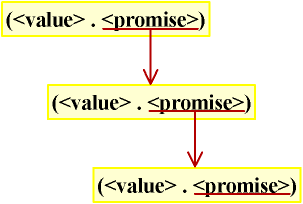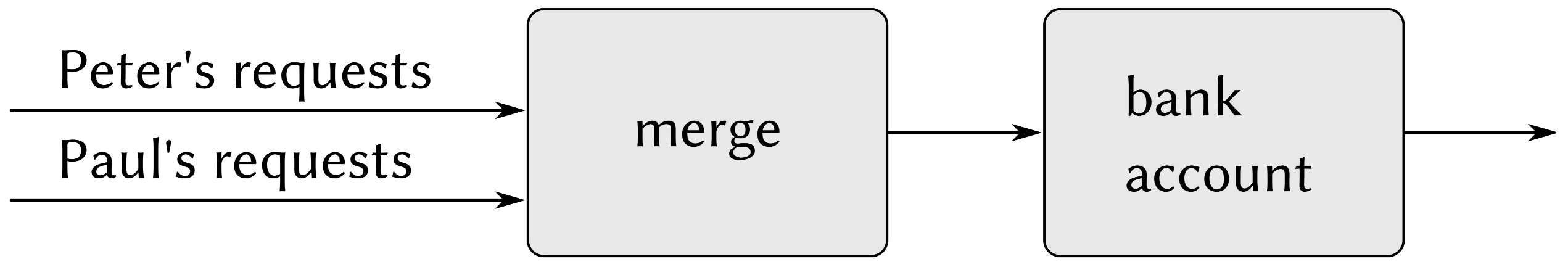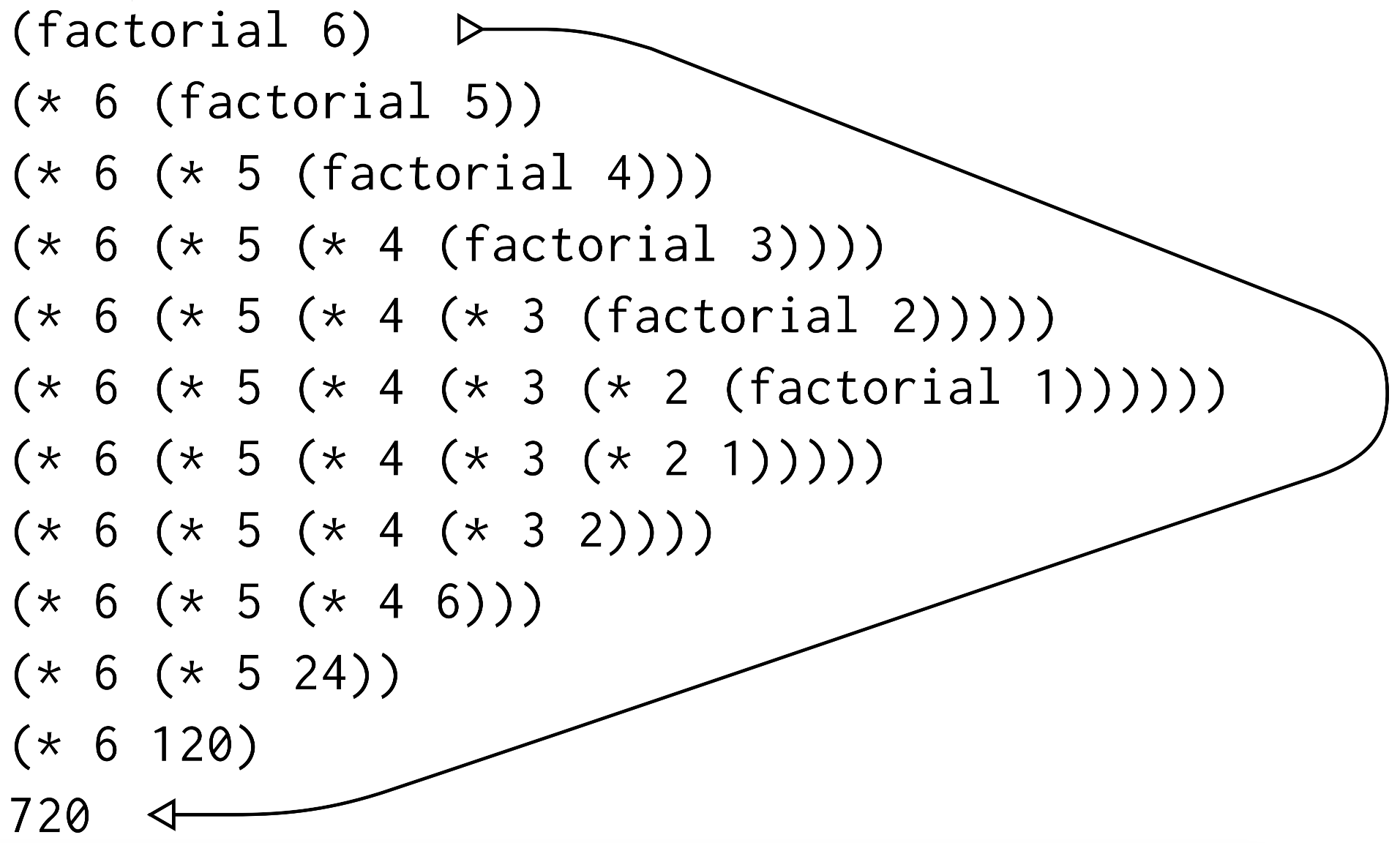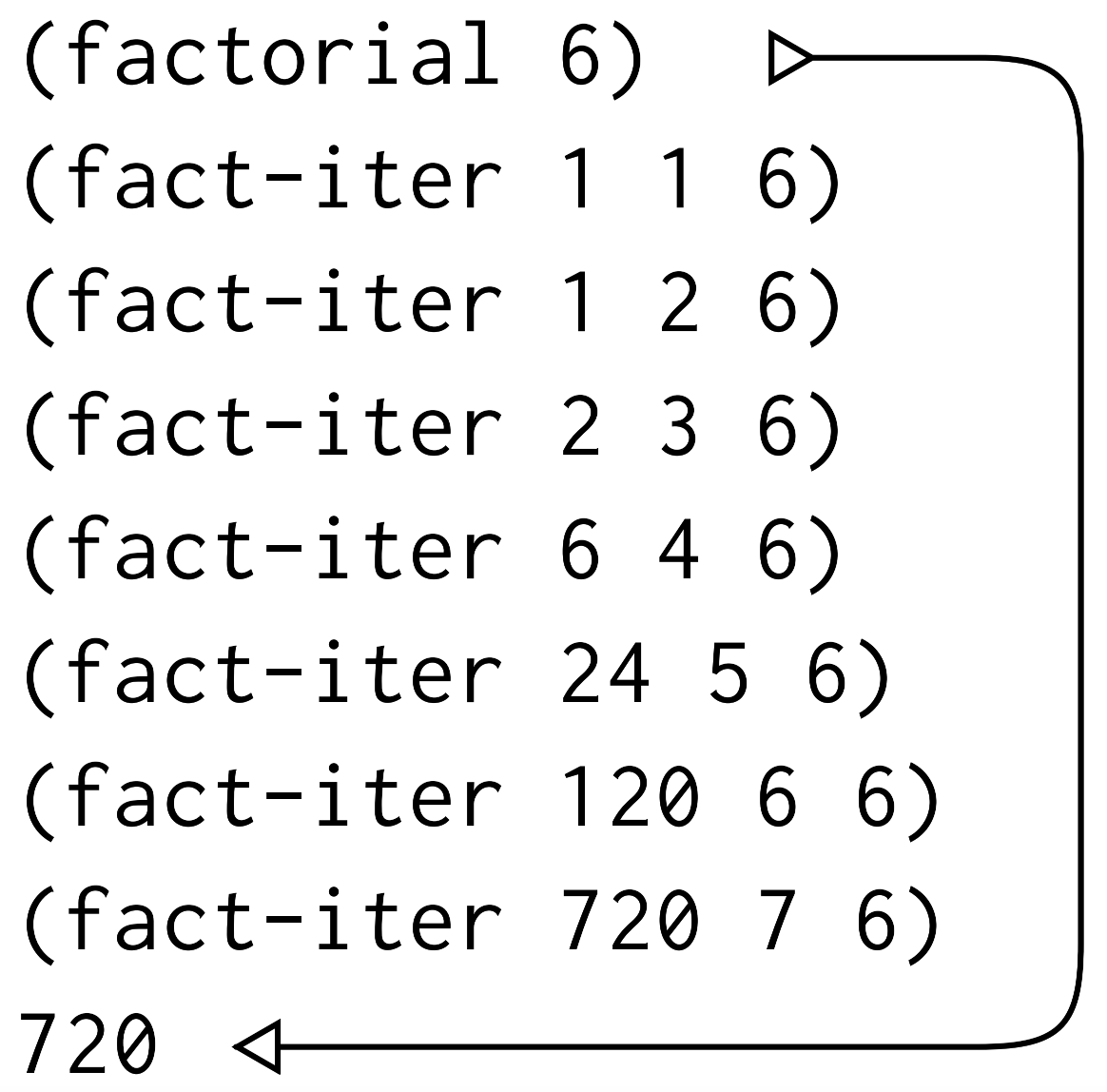——赫拉克利特

——僧肇

# 1 Scheme中的流简介

(define (sum-primes a b)
(define (iter count accum)
(cond ((> count b) accum)
((prime? count) (iter (+ count 1) (+ count accum)))
(else (iter (+ count 1) accum))))
(iter a 0))


(define (sum-primes a b)
(reduce +
(filter prime? (enumerate-interval a b))))


(car (cdr (filter prime?
(enumerate-interval 10000 1000000))))


scm> (equal? (stream-car (cons-stream x y)) x)
#t
scm> (equal? (stream-cdr (cons-stream x y)) y)
#t


scm> (define (stream-enumerate-interval low high)
(if (> low high)
nil
(cons-stream
low
(stream-enumerate-interval (+ low 1) high))))
stream-enumerate-interval
scm> (car (cdr (stream-filter prime?
(stream-enumerate-interval 10000 1000000))))
10009


# 2 惰性求值

：事实上，futurepromisedelaydeferred等来自函数式编程的特性已经被许多语言的并发模块所吸纳。在并发编程中，我们常常会对程序的执行进行同步，而由于某些计算（或者网络请求）尚未结束，我们需要一个对象（也即futurepromise）来代理这个未知的结果。

class Promise:
def __init__(self, expr, env):
self.expr = expr
self.env = env

def __str__(self):
return "#[promise ({0}forced)]".format(
"not " if self.expr is not None else "")


def eval_delay(expr, env):
validate_form(expr, 1, 1)
return Promise(expr.first, env)


delay一起使用的还有一个称为force的基本过程，它以一个延时对象为参数，执行相应的求值工作，也即迫使delay完成它所允诺的求值。

@ primitive("force")
def scheme_force(obj):
from eval_apply import scheme_eval

validate_type(obj, lambda x: is_scheme_promise(x), 0, "stream-force")
return scheme_eval(obj.expr, obj.env)


scm> (define pms1 (delay (+ 2 3)))
pms1
scm> pms1
#[promise (not forced)]
scm> (force pms1)
5
scm> (define pms2 (delay (delay (+ 2 3))))
pms2
scm> (force pms2)
#[promise (not forced)]
scm> (force (force pms2))
5


# 3 流的实现

## 3.1 构造流

def eval_cons_stream(expr, env):
validate_form(expr, 2, 2)
return scheme_cons(scheme_eval(expr.first, env), Promise(expr.rest.first, env))


@primitive("stream-car")
def stream_car(stream):
validate_type(stream, lambda x: is_stream_pair(x), 0, "stream-car")
return stream.first

@primitive("stream-cdr")
def stream_cdr(stream):
validate_type(stream, lambda x: is_stream_pair(x), 0, "stream-cdr")
return scheme_force(stream.rest)


stream-car选取有关序对的first部分，stream-cdr选取有关序对的cdr部分，并求值这里的延时表达式，以获得这个流的剩余部分。

## 3.2 流的行为方式

scm> (define (stream-enumerate-interval low high)
(if (> low high)
nil
(cons-stream
low
(stream-enumerate-interval (+ low 1) high))))
stream-enumerate-interval


scm> (define integers (stream-enumerate-interval 10000 1000000))
integers
scm> integers
(10000 . #[promise (not forced)])


scm> (stream-cdr integers)
(10001 . #[promise (not forced)])
scm> (stream-cdr (stream-cdr integers))
(10002 . #[promise (not forced)])scm> (define (integers-starting-from n)
(cons-stream n (integers-starting-from (+ n 1))))
integers-starting-from
scm> (define integers (integers-starting-from 1))
integers


scm> integers
(1 . #[promise (not forced)])
scm> (stream-cdr integers)
(2 . #[promise (not forced)])
scm> (stream-cdr (stream-cdr integers))
(3 . #[promise (not forced)])
...


## 3.3 针对流的序列操作

stream-map是与过程map类似的针对流的过程，其定义如下：

@primitive("stream-map", use_env=True)
def stream_map(proc, stream, env):
from eval_apply import complete_apply
validate_type(proc, is_scheme_procedure, 0, "map")
validate_type(stream, is_stream_pair, 1, "map")

def stream_map_iter(proc, stream, env):
if is_stream_null(stream):
return nil
return scheme_cons(complete_apply(proc, scheme_list(stream_car(stream)
), env),
stream_map_iter(proc, stream_cdr(stream), env))

return stream_map_iter(proc, stream, env)


stream_map将对流的car应用过程proc，然后需要进一步将过程proc应用于输入流的cdr，这里对stream_cdr的调用将迫使系统对延时的流进行求值。注意，这里我们为了方便延时，使stream_map函数直接返回用scheme_cons函数构造的普通表，在Scheme的实际实现中返回的仍然是流。

@primitive("stream-filter", use_env=True)
def stream_filter(predicate, stream, env):
from eval_apply import complete_apply
validate_type(predicate, is_scheme_procedure, 0, "filter")
validate_type(stream, is_stream_pair, 1, "filter")

def scheme_filter_iter(predicate, stream, env):
if is_stream_null(stream):
return nil
elif complete_apply(predicate, scheme_list(stream_car(stream)), env):
return scheme_cons(stream_car(stream),
scheme_filter_iter(predicate,
stream_cdr(stream), env))
else:
return scheme_filter_iter(predicate, stream_cdr(stream), env)

return scheme_filter_iter(predicate, stream, env)

@primitive("stream-reduce", use_env=True)
def stream_reduce(op, stream, env):
from eval_apply import complete_apply
validate_type(op, is_scheme_procedure, 0, "reduce")
validate_type(stream, lambda x: x is not nil, 1, "reduce")
validate_type(stream, is_stream_pair, 1, "reduce")

def scheme_reduce_iter(op, initial, stream, env):
if is_stream_null(stream):
return initial
return complete_apply(op, scheme_list(stream_car(stream),
scheme_reduce_iter(op,
initial,
stream_cdr(
stream),
env)), env)

return scheme_reduce_iter(op, stream_car(stream), stream_cdr(stream), env)


scm> (stream-map (lambda (x) (* 2 x))  (stream-enumerate-interval 1 10))
(2 4 6 8 10 12 14 16 18 20)


# 4 时间的函数式程序观点

scm> (define (make-simplified-withdraw balance)
(lambda (amount)
(set! balance (- balance amount))
balance))
make-simplified-withdraw
scm> (define W (make-simplified-withdraw 25))
w
scm> (W 20)
5
scm> (W 10)
-5


(define (stream-withdraw balance amount-stream)
(cons-stream
balance
(stream-withdraw (- balance (stream-car amount-stream))
(stream-cdr amount-stream))))


scm> (define amount (cons-stream 20 (cons-stream 10 nil)))
amount
scm> (define W (stream-withdraw 25 amount))
w
scm> (stream-cdr W)
(5 . #[promise (not forced)])
scm> (stream-cdr (stream-cdr W))
(-5 . #[promise (not forced)])• 将这一世界模拟为一集相互分离的、受时间约束的、具有状态的相互交流的对象。

• 将它模拟为单一的、无时间也无状态的统一体（unity）。

# 5 用惰性求值实现尾递归

(define (factorial n)
(if (= n 1)
1
(* n (factorial (- n 1)))))(define (factorial n)
(fact-iter 1 1 n))

(define (fact-iter product counter max-count)
(if (> counter max-count)
product
(fact-iter (* counter product)
(+ counter 1)
max-count)))@primitive("eval", use_env=True)
def scheme_eval(expr, env, _=None):
# Evaluate self-evaluating expressions
if is_self_evaluating(expr):
return expr
# Evaluate variables
elif is_scheme_variable(expr):
return env.lookup_variable_value(expr)

...
# Evaluate special forms
if is_scheme_symbol(first) and first in SPECIAL_FORMS:
return SPECIAL_FORMS[first](rest, env)
# Evaluate an application
else:
operator = scheme_eval(first, env)
# Check if the operator is a macro
if isinstance(operator, MacroProcedure):
return scheme_eval(complete_apply(operator, rest, env), env)
operands = rest.map(lambda x: scheme_eval(x, env))
return scheme_apply(operator, operands, env)


class TailPromise(Promise):
"""An expression and an environment in which it is to be evaluated."""


def optimize_tail_calls(original_scheme_eval):
def optimized_eval(expr, env, tail=False):
# If tail is True and the expression is not variable or self-evaluated,
# return Promise directly, Note that for optimized_eval, argument
# tail defaults to False, which means that it is impossible to
# return Promise at the first call, that is, when the recursion depth
# is 1
if tail and not is_scheme_variable(expr) and not is_self_evaluating(
expr):
return TailPromise(expr, env)

# If tail is False or the expression is variable or self-evaluated (
# which includes the first call of scheme_eval), it will be
# evaluated until the actual value is obtained (instead of Promise)
result = TailPromise(expr, env)
while (isinstance(result, TailPromise)):
# A call to original_scheme_eval actually can simulate the
# recursion depth plus one.
result = original_scheme_eval(result.expr, result.env)
return result

return optimized_eval

# Uncomment the following line to apply tail call optimization
scheme_eval = optimize_tail_calls(scheme_eval)


from functools import wraps
def optimize_tail_calls(original_scheme_eval):
@wraps(original_scheme_eval)
def optimized_eval(expr, env, tail=False):
...
return result

return optimized_eval

@optimize_tail_calls
@primitive("eval", use_env=True)
def scheme_eval(expr, env, _=None):
...


scm> (define (sum n total)
(if (zero? n) total
(sum (- n 1) (+ n total))))
sum
scm> (sum 1000001 0)
500001500001


OK，我们已经实现好了尾递归功能，这依赖于底层惰性求值的实现。但是别忘了，我们有时候是不需要惰性求值，而是需要急切求值的（也即求值结果不能是TailPromise对象）。比如在对MacroProcedure过程对象（该过程对象由宏的定义产生）进行实际应用前，我们需要先将宏的内容进行进行展开，而这就需要我们另外定义一个complete_apply函数：

def complete_apply(procedure, args, env):
val = scheme_apply(procedure, args, env)
if isinstance(val, TailPromise):
return scheme_eval(val.expr, val.env)
else:
return val


if isinstance(operator, MacroProcedure):
return scheme_eval(complete_apply(operator, rest, env), env)


@ primitive("map", use_env=True)
def scheme_map(proc, items, env):
...
def scheme_map_iter(proc, items, env):
if is_scheme_null(items):
return nil
return scheme_cons(scheme_apply(proc, scheme_list(items.first), env),
scheme_map_iter(proc, items.rest, env))

return scheme_map_iter(proc, items, env)


scm> (map (lambda (x) (* 2 x))  (list 1 2 3))
(#[promise (not forced)] #[promise (not forced)] #[promise (not forced)])


@ primitive("map", use_env=True)
def scheme_map(proc, items, env):
...
def scheme_map_iter(proc, items, env):
if is_scheme_null(items):
return nil
return scheme_cons(complete_apply(proc, scheme_list(items.first), env),
scheme_map_iter(proc, items.rest, env))

return scheme_map_iter(proc, items, env)


scm> (map (lambda (x) (* 2 x))  (list 1 2 3))
(2 4 6)


# 参考

•  Abelson H, Sussman G J. Structure and interpretation of computer programs[M]. The MIT Press, 1996.
•  8.6 Lazy evaluation
•  Wiki: Lazy evaluation
•  Yet Another Scheme Tutorial: 17. Lazy evaluation
•  Wiki: Futures and promises
•  Wiki: World line
•  Backus J. Can programming be liberated from the von Neumann style? A functional style and its algebra of programs[J]. Communications of the ACM, 1978, 21(8): 613-641.
•  Dean J, Ghemawat S. MapReduce: simplified data processing on large clusters[J]. Communications of the ACM, 2008, 51(1): 107-113.
•  Zaharia M, Chowdhury M, Das T, et al. Resilient distributed datasets: A fault-tolerant abstraction for in-memory cluster computing[C]//Presented as part of the 9th {USENIX} Symposium on Networked Systems Design and Implementation ({NSDI} 12). 2012: 15-28.
posted @ 2023-05-21 22:14  orion-orion  阅读(220)  评论(0编辑  收藏  举报